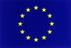## Odabrane teme za radoznale srednjoškolce - Selected Topics for Curious High School Students

Kulcsszavak: Dirichlet Integral, Integration, Convergence, Quantum Computing, Shannon Sampling Theorem, Aliasing
Absztrakt:

In first part of this material we show the convergence and calculate the value of Dirichlet Integral using only the methods of real analysis. As a first step we introduce the concept of indefinite integrals and convergence.  Second, we prove a special case of Riemann–Lebesgue lemma without the use of functional and Fourier analysis. In the end we use Riemann–Lebesgue lemma to calculate the value of the integral. In the second part of this material we introduce the concept of Quantum Computer. We introduce theoretical model of quantum computer, and a concept of quantum algorithm and then we show that quantum Fourier transformation is considerably faster then the fast Fourier transformation on "regular" computer, which has great consequences on the security of bank and military systems. In the third part of this material we introduce concepts of sampling and aliasing. First we review the necessary theory of functional analysis for understanding the proof of Shannon Sampling Theorem. Then we give the proof of the Sampling Theorem and we discus the real life applications of the theorem. This material is aimed to ambitious high school students who wish to explore advanced mathematical concepts, and real life applications of mathematic.

The development is co-financed by the European Union in the fram of the project IPA HU-SRB/1203/221/024: Non-Standard Forms of Teaching Mathematics and PhysicsSzükséges szoftverek: